• DBN深度信念网络
2022-04-07 21:10:42

# 三、DBN

## 3.1 生成模型

深度信念网络是一个生成模型，用来生成符合特定分布的样本。隐变量用来描述在可观测变量之间的高阶相关性。假如加入服从分布 𝑝(𝑣)的训练数据，通过训练得到一个深度信念网络。生成样本时，先在最顶两层进行足够多的吉布斯采样，在达到热平衡时生成样本ℎ^((𝐿−1))，然后依次计算下一层隐变量的分布。因为在给定上一层变量取值时，下一层的变量是条件独立的，故可独立采样。这样，从第𝐿−1层开始，自顶向下进行逐层采样，最终得到可观测层的样本。

## 3.2 参数学习

深度信念网络最直接的训练方式是最大化可观测变量的边际分布𝑝(𝑣)在训练集上的似然 。但是在深度信念网络中，隐变量ℎ之间的关系十分复杂，由于“贡献度分配问题”，很难直接学习。即使对于简单的单层Sigmoid信念网络：

p ( v = 1 ∣ h ) = σ ( b + ω T h ) p(v=1 \mid h)=\sigma\left(b+\omega^{T} h\right)

在已知可观测变量时，其隐变量的联合后验概率𝑝(ℎ|𝑣)不再相互独立，因此很难估计所有隐变量的后验概率，早期深度信念网络的后验概率一般通过蒙特卡洛方法或变分方法来近似估计，但效率低，从而导致其参数学习比较困难。

为了有效训练深度信念网络，我们将每一层的Sigmoid信念网络转换为受限玻尔兹曼机，这样做的好处是隐变量的后验概率事相互独立的，从而可容易进行采样。这样，深度信念网络可看做多个受限玻尔兹曼机从下到上进行堆叠，第𝑙层受限玻尔兹曼机的隐层作为第𝑙+1受限玻尔兹曼机的可观测层。进一步，深度信念网络可采用逐层训练的方式来快速训练，即从最底层开始，每次只训练一层，直到最后一层。

深度信念网络的训练过程可分为逐层预训练和精调两个阶段，先通过逐层预训练将模型的参数初始化为较优的值，然后通过传统机器学习方法对参数进行精调。

## 3.2 逐层预训练

采用逐层训练的方式，将深度信念网络的训练简化为对多个受限玻尔兹曼机的训练。具体的逐层训练过程为自下而上依次训练每一层的首先玻尔兹曼机。假设已训练好前𝑙−1层的受限玻尔兹曼机，可计算隐变量自下而上的条件概率：

p ( h ( i ) ∣ h ( i − 1 ) ) = σ ( b i + W ( i ) h ( i − 1 ) ) , 1 ≤ i ≤ ( l − 1 ) p\left(h^{(i)} \mid h^{(i-1)}\right)=\sigma\left(b^{i}+W^{(i)} h^{(i-1)}\right), 1 \leq i \leq(l-1)

这样可按照 𝑣 = h ( 0 ) → ⋯ → h ( 𝑙 − 1 ) 𝑣=ℎ^{(0)}→⋯→ℎ^{(𝑙−1)} 的顺序生成一组 h 𝑙 − 1 ℎ^{𝑙−1} 的样本，记为 H ( l − 1 ) = h ( l , 1 ) , . . . , h ( l , M ) H^{(l-1)} = h^{(l,1)},...,h^{(l,M)} 。然后将 h ( 𝑙 − 1 ) ℎ^{(𝑙−1)} h ( 𝑙 ) ℎ^{(𝑙)} 组成一个受限玻尔兹曼机，用 𝐻 ( 𝑙 − 1 ) 𝐻^{(𝑙−1)} 作为训练集充分训练第𝑙层的受限玻尔兹曼机.大量实践表明，逐层预训练可以产生非常好的参数初始值，从而极大地降低了模型的学习难度。

## 3.3 精调

经过预训练，再结合具体的任务（监督或无监督学习），通过传统的全局学习算法对网络进行精调（fine-tuning），使模型收敛到更好的局部最优点。

作为生成模型的精调：除了顶层的受限玻尔兹曼机，其它层之间的权重可以被分为向上的认知权重（Recognition Weight） 𝑊 ∗ 𝑊^* 和向下的生成权重（Generative Weight）𝑊。认知权重用来计算后验概率，生成权重用来定义模型，认知权重初始值 𝑊(‘𝑙)=𝑊(𝑙)𝑇

深度信念网络一般采用 Contrastive Wake-Sleep算法进行精调：

1.Wake阶段：认知过程，通过外界输入（可观测变量）和向上的认知权重，计算每一层隐变量的后验概率并采样。修改下行的生成权重使得下一层的变量的后验概率最大。

2.Sleep阶段：生成过程，通过顶层的采样和向下的生成权重，逐层计算每一层的后验概率并采样。然后，修改向上的认知权重使得上一层变量的后验概率最大。

3.交替进行Wake和Sleep过程，直到收敛作为判别模型的精调 ：深度信念网络的一个应用是作为深度神经网络的预训练模型，提供神经网络的初始权重，这时只需要向上的认知权重，作为判别模型使用：# 四 代码演示

import sys
import numpy

numpy.seterr(all='ignore')

def sigmoid(x):
return 1. / (1 + numpy.exp(-x))

def softmax(x):
e = numpy.exp(x - numpy.max(x))  # prevent overflow
if e.ndim == 1:
return e / numpy.sum(e, axis=0)
else:
return e / numpy.array([numpy.sum(e, axis=1)]).T  # ndim = 2

class DBN(object):
def __init__(self, input=None, label=None,\
n_ins=2, hidden_layer_sizes=[3, 3], n_outs=2,\
numpy_rng=None):

self.x = input
self.y = label

self.sigmoid_layers = []
self.rbm_layers = []
self.n_layers = len(hidden_layer_sizes)  # = len(self.rbm_layers)

if numpy_rng is None:
numpy_rng = numpy.random.RandomState(1234)

assert self.n_layers > 0

# construct multi-layer
for i in range(self.n_layers):
# layer_size
if i == 0:
input_size = n_ins
else:
input_size = hidden_layer_sizes[i - 1]

# layer_input
if i == 0:
layer_input = self.x
else:
layer_input = self.sigmoid_layers[-1].sample_h_given_v()
# print('=============')

# construct sigmoid_layer
sigmoid_layer = HiddenLayer(input=layer_input,
n_in=input_size,
n_out=hidden_layer_sizes[i],
numpy_rng=numpy_rng,
activation=sigmoid)
self.sigmoid_layers.append(sigmoid_layer)

# construct rbm_layer
rbm_layer = RBM(input=layer_input,
n_visible=input_size,
n_hidden=hidden_layer_sizes[i],
W=sigmoid_layer.W,     # W, b are shared
hbias=sigmoid_layer.b)
self.rbm_layers.append(rbm_layer)

# layer for output using Logistic Regression
self.log_layer = LogisticRegression(input=self.sigmoid_layers[-1].sample_h_given_v(),
label=self.y,
n_in=hidden_layer_sizes[-1],
n_out=n_outs)

# finetune cost: the negative log likelihood of the logistic regression layer
self.finetune_cost = self.log_layer.negative_log_likelihood()

def pretrain(self, lr=0.1, k=1, epochs=100):
# pre-train layer-wise
# print('pre-training')
for i in range(self.n_layers):
if i == 0:
layer_input = self.x
else:
layer_input = self.sigmoid_layers[i-1].sample_h_given_v(layer_input)
# print(layer_input)
rbm = self.rbm_layers[i]

for epoch in range(epochs):
rbm.contrastive_divergence(lr=lr, k=k, input=layer_input)
# cost = rbm.get_reconstruction_cross_entropy()
# print >> sys.stderr, \
#        'Pre-training layer %d, epoch %d, cost ' %(i, epoch), cost

# def pretrain(self, lr=0.1, k=1, epochs=100):
#     # pre-train layer-wise
#     for i in range(self.n_layers):
#         rbm = self.rbm_layers[i]

#         for epoch in range(epochs):
#             layer_input = self.x
#             for j in range(i):
#                 layer_input = self.sigmoid_layers[j].sample_h_given_v(layer_input)

#             rbm.contrastive_divergence(lr=lr, k=k, input=layer_input)
#             # cost = rbm.get_reconstruction_cross_entropy()
#             # print >> sys.stderr, \
#             #        'Pre-training layer %d, epoch %d, cost ' %(i, epoch), cost

def finetune(self, lr=0.1, epochs=100):
# print('finetune')
layer_input = self.sigmoid_layers[-1].sample_h_given_v()

# train log_layer
epoch = 0
done_looping = False
while (epoch < epochs) and (not done_looping):
self.log_layer.train(lr=lr, input=layer_input)
# self.finetune_cost = self.log_layer.negative_log_likelihood()
# print >> sys.stderr, 'Training epoch %d, cost is ' % epoch, self.finetune_cost

lr *= 0.95
epoch += 1

def predict(self, x):
layer_input = x

for i in range(self.n_layers):
sigmoid_layer = self.sigmoid_layers[i]
# rbm_layer = self.rbm_layers[i]
layer_input = sigmoid_layer.output(input=layer_input)

out = self.log_layer.predict(layer_input)
return out

class HiddenLayer(object):
def __init__(self, input, n_in, n_out,\
W=None, b=None, numpy_rng=None, activation=numpy.tanh):

if numpy_rng is None:
numpy_rng = numpy.random.RandomState(1234)

if W is None:
a = 1. / n_in
initial_W = numpy.array(numpy_rng.uniform(  # initialize W uniformly
low=-a,
high=a,
size=(n_in, n_out)))

W = initial_W

if b is None:
b = numpy.zeros(n_out)  # initialize bias 0

self.numpy_rng = numpy_rng
self.input = input
self.W = W
self.b = b

self.activation = activation

def output(self, input=None):
if input is not None:
self.input = input

linear_output = numpy.dot(self.input, self.W) + self.b

return (linear_output if self.activation is None
else self.activation(linear_output))

def sample_h_given_v(self, input=None):
if input is not None:
self.input = input

v_mean = self.output()
# print('v_mean:\n',v_mean)
h_sample = self.numpy_rng.binomial(size=v_mean.shape,
n=1,
p=v_mean)
# print('h_sample:\n',h_sample)
return h_sample

class RBM(object):
def __init__(self, input=None, n_visible=2, n_hidden=3, \
W=None, hbias=None, vbias=None, numpy_rng=None):

self.n_visible = n_visible  # num of units in visible (input) layer
self.n_hidden = n_hidden    # num of units in hidden layer

if numpy_rng is None:
numpy_rng = numpy.random.RandomState(1234) # 使用RandomState获得随机数生成器。

if W is None:
a = 1. / n_visible
initial_W = numpy.array(numpy_rng.uniform(  # initialize W uniformly
low=-a,
high=a,
size=(n_visible, n_hidden)))  # 生成权重矩阵

W = initial_W

if hbias is None:
hbias = numpy.zeros(n_hidden)  # initialize h bias 0

if vbias is None:
vbias = numpy.zeros(n_visible)  # initialize v bias 0

self.numpy_rng = numpy_rng
self.input = input
self.W = W
self.hbias = hbias
self.vbias = vbias
# self.params = [self.W, self.hbias, self.vbias]

def contrastive_divergence(self, lr=0.1, k=1, input=None):
if input is not None:
self.input = input

''' CD-k '''
ph_mean, ph_sample = self.sample_h_given_v(self.input)

chain_start = ph_sample

for step in range(k):
if step == 0:
nv_means, nv_samples,\
nh_means, nh_samples = self.gibbs_hvh(chain_start)
else:
nv_means, nv_samples,\
nh_means, nh_samples = self.gibbs_hvh(nh_samples)
# nv_means, nv_samples, nh_means, nh_samples = self.gibbs_hvh(chain_start)
# for _ in range(1, k):
#         nv_means, nv_samples, nh_means, nh_samples = self.gibbs_hvh(nh_samples)

# chain_end = nv_samples

self.W += lr * (numpy.dot(self.input.T, ph_sample)
- numpy.dot(nv_samples.T, nh_means))
self.vbias += lr * numpy.mean(self.input - nv_samples, axis=0)
self.hbias += lr * numpy.mean(ph_sample - nh_means, axis=0)

# cost = self.get_reconstruction_cross_entropy()
# return cost

def sample_h_given_v(self, v0_sample):
h1_mean = self.propup(v0_sample)  #
h1_sample = self.numpy_rng.binomial(size=h1_mean.shape,   # discrete: binomial 二项分布
n=1,
p=h1_mean)

return [h1_mean, h1_sample]

def sample_v_given_h(self, h0_sample):
v1_mean = self.propdown(h0_sample)
v1_sample = self.numpy_rng.binomial(size=v1_mean.shape,   # discrete: binomial
n=1,
p=v1_mean)
return [v1_mean, v1_sample]

def propup(self, v):
# 返回隐藏层被激活的概率，应该是一个矩阵，对应的是每一个神经元被激活的概率
pre_sigmoid_activation = numpy.dot(v, self.W) + self.hbias
return sigmoid(pre_sigmoid_activation)

def propdown(self, h):
pre_sigmoid_activation = numpy.dot(h, self.W.T) + self.vbias
return sigmoid(pre_sigmoid_activation)

def gibbs_hvh(self, h0_sample):
v1_mean, v1_sample = self.sample_v_given_h(h0_sample)
h1_mean, h1_sample = self.sample_h_given_v(v1_sample)
return [v1_mean, v1_sample, h1_mean, h1_sample]

def get_reconstruction_cross_entropy(self):
pre_sigmoid_activation_h = numpy.dot(self.input, self.W) + self.hbias
sigmoid_activation_h = sigmoid(pre_sigmoid_activation_h)

pre_sigmoid_activation_v = numpy.dot(sigmoid_activation_h, self.W.T) + self.vbias
sigmoid_activation_v = sigmoid(pre_sigmoid_activation_v)

cross_entropy =  - numpy.mean(
numpy.sum(self.input * numpy.log(sigmoid_activation_v) +
(1 - self.input) * numpy.log(1 - sigmoid_activation_v),
axis=1))

return cross_entropy

def reconstruct(self, v):
h = sigmoid(numpy.dot(v, self.W) + self.hbias)
reconstructed_v = sigmoid(numpy.dot(h, self.W.T) + self.vbias)
return reconstructed_v

class LogisticRegression(object):
def __init__(self, input, label, n_in, n_out):
self.x = input
self.y = label
self.W = numpy.zeros((n_in, n_out))  # initialize W 0
self.b = numpy.zeros(n_out)          # initialize bias 0

def train(self, lr=0.1, input=None, L2_reg=0.00):
if input is not None:
self.x = input

p_y_given_x = softmax(numpy.dot(self.x, self.W) + self.b)
d_y = self.y - p_y_given_x

self.W += lr * numpy.dot(self.x.T, d_y) - lr * L2_reg * self.W
self.b += lr * numpy.mean(d_y, axis=0)

def negative_log_likelihood(self):
sigmoid_activation = softmax(numpy.dot(self.x, self.W) + self.b)

cross_entropy = - numpy.mean(
numpy.sum(self.y * numpy.log(sigmoid_activation) +
(1 - self.y) * numpy.log(1 - sigmoid_activation),
axis=1))

return cross_entropy

def predict(self, x):
return softmax(numpy.dot(x, self.W) + self.b)

def test_dbn(pretrain_lr=0.1, pretraining_epochs=1000, k=1, \
finetune_lr=0.1, finetune_epochs=200):

x = numpy.array([[1,1,1,0,0,0],
[1,0,1,0,0,0],
[1,1,1,0,0,0],
[0,0,1,1,1,0],
[0,0,1,1,0,0],
[0,0,1,1,1,0]])
y = numpy.array([[1, 0],
[1, 0],
[1, 0],
[0, 1],
[0, 1],
[0, 1]])

rng = numpy.random.RandomState(123)

# construct DBN
# print('construct DBN')
dbn = DBN(input=x, label=y, n_ins=6, hidden_layer_sizes=[3, 3], n_outs=2, numpy_rng=rng)

# pre-training (TrainUnsupervisedDBN)
# k是gibbs的次数

dbn.pretrain(lr=pretrain_lr, k=1, epochs=pretraining_epochs)

# fine-tuning (DBNSupervisedFineTuning)
dbn.finetune(lr=finetune_lr, epochs=finetune_epochs)

# test
x = numpy.array([1, 1, 0, 0, 0, 0])
print(dbn.predict(x))

if __name__ == "__main__":
test_dbn()

[0.72344411 0.27655589]机器学习
更多相关内容
• Matlab编写的深度学习，DBN深度信念神经网络
• 深度信念网络回归算法，内含受限玻尔兹曼机训练、BP微调拟合，最终完成深度信念网络回归算法的训练。算法以Python语言完成，包含多个子文件，全部写入该word文档。
• 深度信念（置信）网络（DBN），使用python开发环境，代码思路清晰，易调试。有问题可以留言相互交流
• 自己编写的深度信念网络模型程序，可以直接调用。本人用DBN进行了光伏发电预测，效果很好。
• 这是一篇题为“使用专用硬件架构计算深度信念网络”的论文的补充信息的一部分。 发表于 IEEE IJCNN 2014 会议。 http://ieeexplore.ieee.org/xpl/login.jsp?tp=&arnumber=6889903 在机器学习中，DBN 是一种生成图...matlab
• 深度信念网络程序详解，可以实现数据回归和分类，自动提取关键成分。
• 传统的深度信念网络规模大、难度大、训练时间长，导致其故障诊断的时间较长。针对该问题，提出了一种基于贝叶斯正则化深度信念网络的电力变压器故障诊断方法。采用贝叶斯正则化算法改进传统深度信念网络的训练性能...
• Deep Belief Network深度信念网络（DBN的Matlab代码），可以运行test_example_DBN.m对手写数字进行训练学习
• 基于多级纹理特征的深度信念网络人脸识别算法.pdf
• 深度信念网络matlab代码 深度信念网络，有代码，有实例，有数据。 用于深度网络预训练。
• 深度信念网络分类算法，内含受限玻尔兹曼机训练、BP分类微调拟合，最终完成深度信念网络分类算法的训练。算法以Python语言完成，包含多个子文件，全部写入该word文档。文档注释详细，适合学习。
• 深度信念网络 (Deep Belief Network, DBN) 由 Geoffrey Hinton 在 2006 年提出。它是一种生成模型，通过训练其神经元间的权重，我们可以让整个神经网络按照最大概率来生成训练数据。我们不仅可以使用 DBN 识别特征、...人工智能
• 基于DBN-ELM深度信念网络的在线序贯极限学习机的数据分类仿真，matlab2021a仿真测试
• 深度学习是学习样本数据的内在规律和表示层次，这些学习过程中获得的信息对诸如文字，图像和声音等数据的解释有很大的帮助。它的最终目标是让机器能够像人一样具有分析学习能力，能够...本资源是对深度信念网络的介绍。
• ## 深度信念网络matlab代码

千次下载 热门讨论 2014-09-03 10:31:12
深度信念网络，有代码，有实例，有数据。 用于深度网络预训练。
• 提出了一种基于深度信念网络（DBN）的基于单通道脑电图的心理疲劳检测方法。 从指定的子带和动态分析中融合了非线性特征，总共提取了21个特征作为DBN的输入，以区分三类心理状态，包括警觉，轻度疲劳和严重疲劳。 ...
• 本篇非常简要地介绍了深度信念网络的基本概念。文章先简要介绍了深度信念网络（包括其应用实例）。接着分别讲述了：(1) 其基本组成结构——受限玻尔兹曼机的的基本情况，以及，(2) 这个基本结构如何组成深度信念网络...
• 神经网络自20世纪50年代发展起来后，因其良好的非线性能力、泛化能力而备受关注。然而，传统的神经网络仍存在一些局限，在上个世纪90年代陷入衰落，主要有以下几个原因： 1、传统的神经网络一般都是单隐层，最多两...

神经网络自20世纪50年代发展起来后，因其良好的非线性能力、泛化能力而备受关注。然而，传统的神经网络仍存在一些局限，在上个世纪90年代陷入衰落，主要有以下几个原因：
1、传统的神经网络一般都是单隐层，最多两个隐层，因为一旦神经元个数太多、隐层太多，模型的参数数量迅速增长，模型训练的时间非常之久；
2、传统的神经网络，随着层数的增加，采用随机梯度下降的话一般很难找到最优解，容易陷入局部最优解。在反向传播过程中也容易出现梯度弥散或梯度饱和的情况，导致模型结果不理想；
3、随着神经网络层数的增加，深度神经网络的模型参数很多，就要求在训练时需要有很大的标签数据，因为训练数据少的时候很难找到最优解，也就是说深度神经网络不具备解决小样本问题的能力。

由于以上的限制，深度的神经网络一度被认为是无法训练的，从而使神经网络的发展一度停滞不前。

2006年，“神经网络之父”Geoffrey Hinton祭出神器，一举解决了深层神经网络的训练问题，推动了深度学习的快速发展，开创了人工智能的新局面，使近几年来科技界涌现出了很多智能化产品，深深地影响了我们每个人的生活。

那这个神器是什么呢？那就是“深度信念网络”（Deep Belief Network，简称DBN）。

深度信念网络，DBN，Deep Belief Nets，神经网络的一种。既可以用于非监督学习，类似于一个自编码机；也可以用于监督学习，作为分类器来使用。
从非监督学习来讲，其目的是尽可能地保留原始特征的特点，同时降低特征的维度。从监督学习来讲，其目的在于使得分类错误率尽可能地小。而不论是监督学习还是非监督学习，DBN的本质都是Feature Learning的过程，即如何得到更好的特征表达。
深度信念网络（DBN）通过采用逐层训练的方式，解决了深层次神经网络的优化问题，通过逐层训练为整个网络赋予了较好的初始权值，使得网络只要经过微调就可以达到最优解。而在逐层训练的时候起到最重要作用的是“受限玻尔兹曼机”（Restricted Boltzmann Machines，简称RBM），为什么叫“受限玻尔兹曼机”呢？因为还有一个是不受限的，那就是“玻尔兹曼机”（Boltzmann Machines，简称BM）。
下面依次介绍一下什么是“玻尔兹曼机”（BM）、“受限玻尔兹曼机”（RBM）？

## 一、玻尔兹曼机（Boltzmann Machines，简称BM）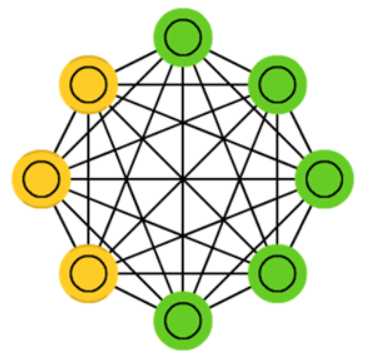玻尔兹曼机于1986年由大神Hinton提出，是一种根植于统计力学的随机神经网络，这种网络中神经元只有两种状态（未激活、激活），用二进制0、1表示，状态的取值根据概率统计法则决定。
由于这种概率统计法则的表达形式与著名统计力学家L.E.Boltzmann提出的玻尔兹曼分布类似，故将这种网络取名为“玻尔兹曼机”。
在物理学上，玻尔兹曼分布（也称为吉布斯分布，Gibbs Distribution）是描述理想气体在受保守外力的作用（或保守外力的作用不可忽略）时，处于热平衡态下的气体分子按能量的分布规律。
在统计学习中，如果我们将需要学习的模型看成高温物体，将学习的过程看成一个降温达到热平衡的过程（热平衡在物理学领域通常指温度在时间或空间上的稳定），最终模型的能量将会收敛为一个分布，在全局极小能量上下波动，这个过程称为“模拟退火”，其名字来自冶金学的专有名词“退火”，即将材料加热后再以一定的速度退火冷却，可以减少晶格中的缺陷，而模型能量收敛到的分布即为玻尔兹曼分布。
听起来很难理解的样子，只需要记住一个关键点：能量收敛到最小后，热平衡趋于稳定，也就是说，在能量最少的时候，网络最稳定，此时网络最优。

玻尔兹曼机（BM）是由随机神经元全连接组成的反馈神经网络，且对称连接，由可见层、隐层组成，BM可以看做是一个无向图，如下图所示：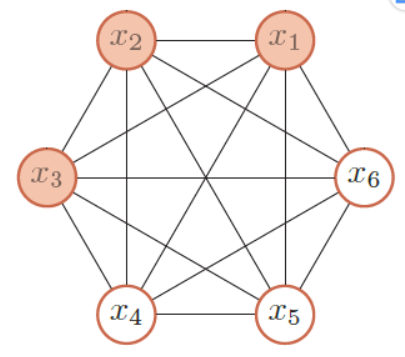其中，x1、x2、x3为可见层，x4、x5、x6为隐层。
整个能量函数定义为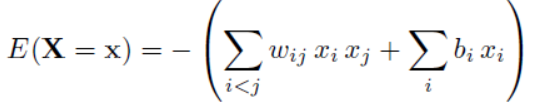其中，w为权重，b为偏置变量，x只有{0,1}两种状态。
根据玻尔兹曼分布，给出的一个系统在特定状态能量和系统温度下的概率分布，如下：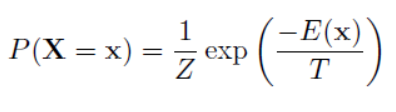前面讲过，“能量收敛到最小后，热平衡趋于稳定”，因此：
1、简单粗暴法
要寻找一个变量使得整个网络的能量最小，一个简单（但是低效）的做法是选择一个变量，在其它变量保持不变的情况下，将这个变量设为会导致整个网络能量更低的状态。那么一个变量Xi的两个状态0（关闭）和1（打开）之间的能量差异为：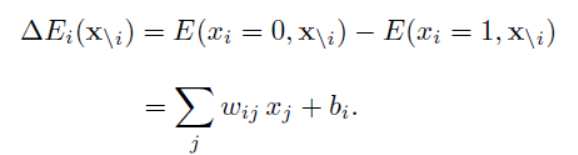这时，如果能量差异ΔE大于一定的阈值（比如0），我们就设Xi = 1（也即取能量小的），否则就设Xi = 0。这种简单的方法通过反复不断运行，在一定时间之后收敛到一个解（可能是局部最优解）。
2、最大似然法
利用“模拟退火”原理寻找全局最优解，根据玻尔兹曼分布，Xi=1的概率为：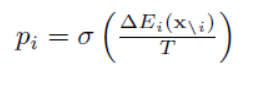训练集v的对数似然函数为：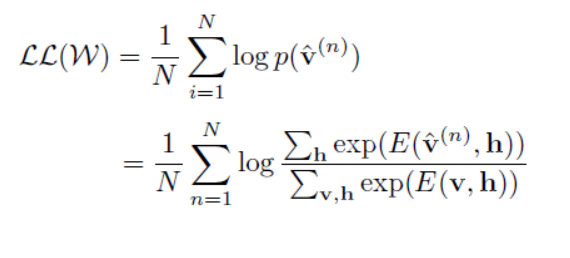对每个训练向量p(v)的对数似然对参数w求导数，得到梯度：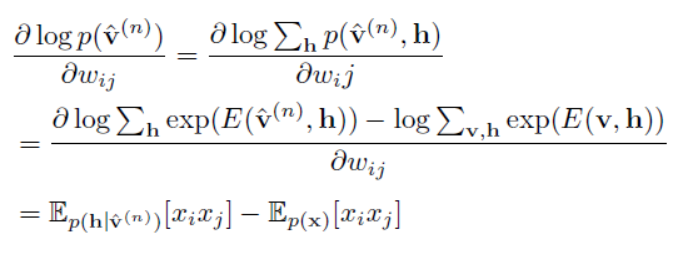跟传统的神经网络类似，参数w的更新公式如下（a为学习率）：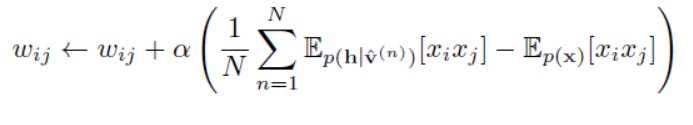好了好了，公式就讲到这里了，看上去挺复杂的，没错，确实计算很复杂，这个梯度很难精确计算，整个计算过程会十分地耗时。
目前，可以通过一些采样方法（例如Gibbs采样）来进行近似求解。

玻尔兹曼机（BM）可以用在监督学习和无监督学习中。在监督学习中，可见变量又可以分为输入和输出变量，隐变量则隐式地描述了可见变量之间复杂的约束关系。在无监督学习中，隐变量可以看做是可见变量的内部特征表示，能够学习数据中复杂的规则。玻尔兹曼机代价是训练时间很长很长很长。

## 二、受限玻尔兹曼机（Restricted Boltzmann Machines，简称RBM）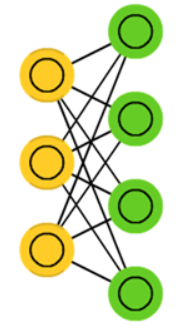所谓“受限玻尔兹曼机”（RBM）就是对“玻尔兹曼机”（BM）进行简化，使玻尔兹曼机更容易更加简单使用，原本玻尔兹曼机的可见元和隐元之间是全连接的，而且隐元和隐元之间也是全连接的，这样就增加了计算量和计算难度。
“受限玻尔兹曼机”（RBM）同样具有一个可见层，一个隐层，但层内无连接，层与层之间全连接，节点变量仍然取值为0或1，是一个二分图。也就是将“玻尔兹曼机”（BM）的层内连接去掉，对连接进行限制，就变成了“受限玻尔兹曼机”（RBM），这样就使得计算量大大减小，使用起来也就方便了很多。如上图。
“受限玻尔兹曼机”（RBM）的特点是：在给定可见层单元状态（输入数据）时，各隐层单元的激活条件是独立的（层内无连接），同样，在给定隐层单元状态时，可见层单元的激活条件也是独立的。

跟“玻尔兹曼机”（BM）类似，根据玻尔兹曼分布，可见层（变量为v，偏置量为a）、隐层（变量为h，偏置量为b）的概率为：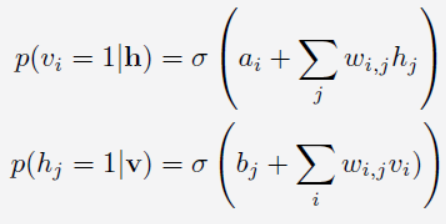训练样本的对数似然函数为：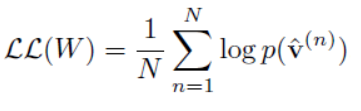求导数：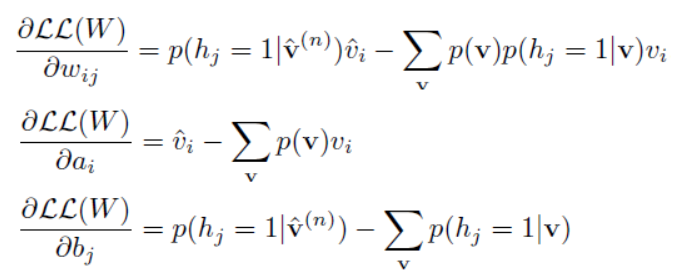总之，还是挺复杂的，计算也还是挺花时间的。
同样，可以通过Gibbs 采样的方法来近似计算。虽然比一般的玻尔兹曼机速度有很大提高，但一般还是需要通过很多步采样才可以采集到符合真实分布的样本。这就使得受限玻尔兹曼机的训练效率仍然不高。
2002年，大神Hinton再出手，提出了“对比散度”（Contrastive Divergence，简称CD）算法，这是一种比Gibbs采样更加有效的学习算法，促使大家对RBM的关注和研究。

RBM的本质是非监督学习的利器，可以用于降维（隐层设置少一点）、学习提取特征（隐层输出就是特征）、自编码器（AutoEncoder）以及深度信念网络（多个RBM堆叠而成）等等。

## 三、深度信念网络（Deep Belief Network，简称DBN）

使用BP算法单独训练每一层的时候，我们发现，必须丢掉网络的第三层，才能级联自联想神经网络。然而，有一种更好的神经网络模型，这就是受限玻尔兹曼机。使用层叠波尔兹曼机组成深度神经网络的方法，在深度学习里被称作深度信念网络DBN，，这是目前非常流行的方法。下面的术语，将把自联想网络称作自编码网络autoencoder.。通过层叠自编码网络的深度网络在深度学习里另外一个属于叫栈式自编码网络。经典的DBN网络结构 是由若干层 RBM 和一层 BP 组成的一种深层神经网络, 结构如下图所示.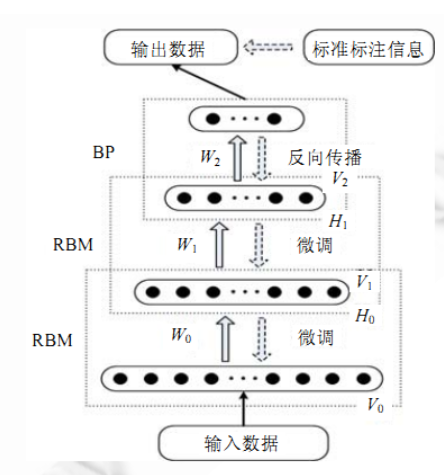DBN 在训练模型的过程中主要分为两步:

第 1 步：分别单独无监督地训练每一层 RBM 网络,确保特征向量映射到不同特征空间时,都尽可能多地保留特征信息;(学习特征)

第 2 步：在 DBN 的最后一层设置 BP 网络,接收 RBM 的输出特征向量作为它的输入特征向量,有监督地训练实体关系分类器.而且每一层 RBM 网络只能确保自身层内的 权值对该层特征向量映射达到最优,并不是对整个 DBN 的特征向量映射达到最优,所以反向传播网络还将错误信息自顶向下传播至每一层 RBM,微调整个 DBN 网络.RBM 网络训练模型的过程可以看作对一个深层 BP 网络权值参数的初始化,使DBN 克服了 BP 网络因随机初始化权值参数而容易陷入局部最优和训练时间长的缺点。

上述训练模型中第一步在深度学习的术语叫做预训练，第二步叫做微调。最上面有监督学习的那一层，根据具体的应用领域可以换成任何分类器模型，而不必是BP网络。

一个DBN模型由若干个RBM堆叠而成，训练过程由低到高逐层进行训练，如下图所示：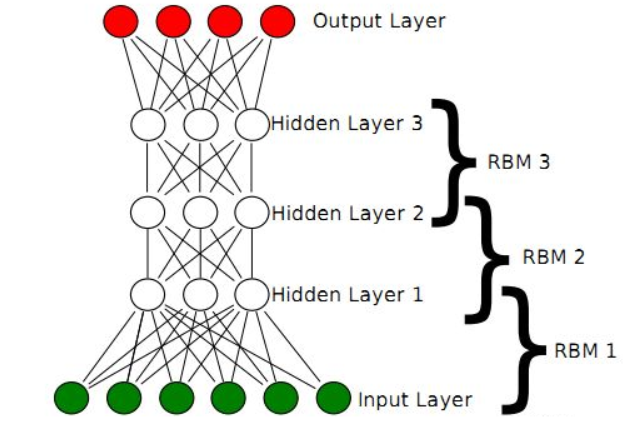展开全文• DBN_；DBN_nan_深度信念_dbn回归_深度信念网络_源码.zip
• 深度信念网络模型（DBN）可以从文本预处理后的特征表示中学习到更具有本质含义的特征表示，便于分类器分类，且避免以上模型的不足。在人工服务语音文本化后，通过深度信念网络模型转换特征表示再进行分类，最终的...
• DBN实例1深度信念网络,dbn深度置信网络,matlab源码
• 深度信念网络，数据实例代码一应俱全。 用于深度网络预训练
• 针对语音情感识别中的特征提取问题,通过多层深度信念网络(DBN)自动提取语音信号中的情感特征,把连续多帧的语音拼接在一起,形成一个高维抽象特征,将深度信念网络训练好的特征作为极限学习机(ELM)分类器的输入端,最终...
• 为此提出一种基于改进深度信念网络的心血管疾病预测模型，利用重构误差，自主确定网络深度，结合无监督训练和有监督调优，在提高模型预测准确率的同时保证稳定性。对UCI数据库中的statlog（heart）和heart disease ......